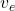A rocket sled with an initial mass of 3 metric tons, invluding 1 ton of fuel, rests on a level section of track. At t=0, the solid fuel of t

Question

A rocket sled with an initial mass of 3 metric tons, invluding 1 ton of fuel, rests on a level section of track. At t=0, the solid fuel of the rocket is ignited and the rocket burns fuel at the rate of 75 kg/s. The exit speed of the exhaust gas relative to the rocket is 2,500 m/s, and the pressure is atmospheric. Neglecting friction and air resistance, calculate the acceleration and speed of the sled at t = 10 s.

in progress 0
6 months 2021-07-15T04:07:25+00:00 1 Answers 4 views 0

v = 719.2 m / s and     a = 83.33 m / s²

Explanation:

This is a rocket propulsion system where the system is made up of the rocket plus the ejected mass, where the final velocity is

v – v₀ =ln (M₀ / M)

where v₀ is the initial velocity, v_{e} the velocity of the gases with respect to the rocket and M₀ and M the initial and final masses of the rocket

In this case, if fuel burns at 75 kg / s, we can calculate the fuel burned for the 10 s

m_fuel = 75 10

m_fuel = 750 kg

As the rocket initially had a mass of 3000 kg including 1000 kg of fuel, there are still 250 kg, so the mass of the rocket minus the fuel burned is

M = 3000 -750 = 2250 kg

let’s calculate

v – 0 = 2500 ln (3000/2250)

v = 719.2 m / s

To calculate the acceleration, let’s use the concept of the rocket thrust, which is the force of the gases on it. In the case of the rocket, it is

Push = v_{e} dM / dt

let’s calculate

Push = 2500  75

Push = 187500 N

If we use Newton’s second law

F = m a

a = F / m

let’s calculate

a = 187500/2250

a = 83.33 m / s²